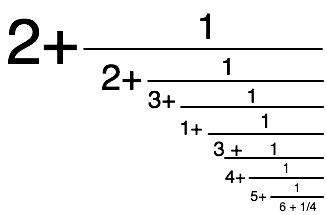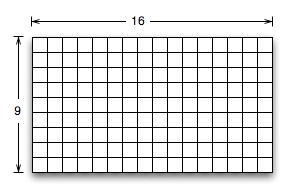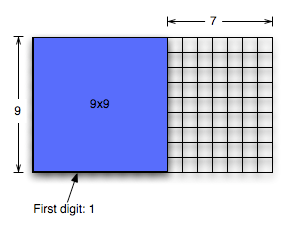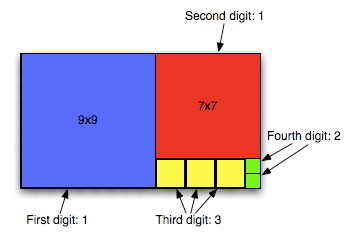# Continued Fractions (classic repost)

I’m away on vacation this week, taking my kids to Disney World. Since I’m not likely to have time to write while I’m away, I’m taking the opportunity to re-run an old classic series of posts on numbers, which were first posted in the summer of 2006. These posts are mildly revised.

One of the annoying things about how we write numbers is the fact that we generally write things one of two ways: as fractions, or as decimals.

You might want to ask, “Why is that annoying?” (And in fact, that’s what I want you to ask, or else there’s no point in my writing the rest of this!)

It’s annoying because both fractions and decimals can both only describe rational numbers – that is, numbers that are a perfect ratio of two integers. The problem with that is that most numbers aren’t rational. Our standard notations are incapable of representing the precise values of the overwhelming majority of numbers!

But it’s even more annoying than that: if you use decimals, then there are lots of rational numbers that you can’t represent exactly (i.e., 1/3); and if you use fractions, then it’s hard to express the idea that the fraction isn’t exact. (How do you write π as a fraction? 22/7 is a standard fractional approximation, but how do you say π, which is almost 22/7?)

So what do we do?

One of the answers is something called continued fractions. A continued fraction is a very neat thing. Here’s the idea: take a number where you don’t know it’s fractional form. Pick the nearest simple fraction 1/n that’s just a little bit too large. If you were looking at, say, 0.4, you’d take 1/2 – it’s a bit bigger. Now – you’ve got an approximation, but it’s too large. So that means that the demoninator is too small. So you add a correction to the denominator to make it a little bit bigger. And you just keep doing that – you approximate the correction to the denominator by adding a fraction to the denominator that’s just a little too big, and then you add a correction to that correction.

Let’s look at an example: 2.3456.

1. It’s close to 2. So we start with 2 + (0.3456).
2. Now, we start approximating the fraction. The way we do that is we take the reciprocal of 0.3456 and take the integer part of it: 1/0.3456 rounded down is 2 . So we make it 2 + 1/2; and we know that the denominator is off by 0.3088.
3. We take the reciprocal again, and get 3, and it’s off by 0.736.
4. We take the reciprocal again, and get 1, and it’s off by 0.264.
5. Next we get 3, but it’s off by 208/1000.
6. Then 4, off by 0.168.
7. Then 5, off by 0.16.
8. Then 6, off by 0.25.
9. Then 4, off by 0; so now we have an exact result.

So as a continued fraction, 2.3456 looks like:As a shorthand, continued fractions are normally written using a list notation inside of square brackets: the integer part, following by a semicolon, followed by a comma-separated list of the denominators of each of the fractions. So our continued fraction for 2.3456 would be written [2; 2, 3, 1, 3, 4, 5, 6, 4].There’s a very cool visual way of understanding that algorithm. I’m not going to show it for 2.3456, because it’s a bit too complicated – it would be hard to draw out the complete diagram for in a legible way. So instead, we’ll look at a simpler number to make the visual work. Let’s write 9/16ths as a continued fraction. Basically, we make a grid consisting of 16 squares across by 9 squares up and down, like this one.We draw the largest square we can on that grid. The number of squares of that size that we can draw is the first digit of the continued fraction. For 9/16ths, we can draw one 9×9 square – so our first digit is one, and we’re left with a 7×9 rectangle:

Now we just repeat: draw the largest square we can. That’s a 7×7, and we can only draw one of it. So the second digit is one; and we’re left with a 7×2:And repeat: the largest square we can draw is a 2×2, and we can draw three of them. So the next digit is a three; and we’re left with 1×2 – which is 2 1×1, so the last digit is a 2.So the continued fraction for 9/16ths is 1/(1+1/(1+3/(1+2))), or [0; 1, 1, 3, 2].

One incredibly nifty thing about this way of writing numbers is: what’s the reciprocal of 2.3456, aka [2; 2, 3, 1, 3, 4, 5, 6, 4]? It’s [0; 2, 2, 3, 1, 3, 4, 5, 6, 4]. We just add a zero to the front as the integer part, and push everything else one place to the right. If it was a zero in front, then we would have removed the zero and pulled everything else one place to the left.

Using continued fractions, we can represent any rational number in a finite-length continued fraction. We still can’t directly represent irrational numbers – they’re going to be infinite continued fractions. But we can usually write some expression to show how to continue the number, and for a given precision, we can approximate irrational numbers with a much smaller representation.

So, as I just said, we represent irrational numbers using infinite continued fractions. So there’s an infinite series of correction fractions. You can understand it as a series of every-improving approximations of the value of the number. And you can define it using a recurrence relation (that is, a recursive formula) for how to get to the next digit – that recurrence relation is the real key – we can include a simple equation that describes how, given the current finite continued fraction, to generate the next step.

For example, π = [3; 7, 15, 1, 292, 1, …]. If we work that out, the first six places of the continued fraction for pi work out in decimal form to 3.14159265392. That’s correct to the first 11 places in decimal. So 9 numerals of continued fractions gives us 11 decimal places. In general, the conciseness savings of continued fractions is even better than that.

I’ll close with a very cool property of continued fractions. The square root of most numbers (excepting the perfect squares) is irrational. In continued fractions, they’re still irrational – that is, they’re infinite continued fractions. But all irrational square roots form repeating continued fractions. The coolest example of this? The square root of 2 in continued fractions is [1; 2, 2, 2, 2, …].

## 0 thoughts on “Continued Fractions (classic repost)”

1.Marcelo

Following the procedure that you described, I get [2; 2, 1, 8, 2, 1, 1, 4]. Adding all the fractions I get 2+216/625, which is indeed 2.3456.
In particular I can’t follow this sentence:
“So we make it 2 + 1/2; and we know that the denominator is off by 0.3088.”
0.3088 wrt what? 0.3456 = 1/(2+x) and solving for x that’s 0.893, not 0.3088 … what am I missing?
Put in another way, 0.3456 = 216/625 = 1/(2+x) (yes, I know, this defeats the whole purpose of the exercise), so x = 193/216.

2.Marcelo

Also, the continued fraction you presented simplifies down to 2+8631/19555

3.Samuel A. Falvo II

An interesting property: it looks as though [0; 0, n1, n2, …] = [n1; n2, …] too, since 1/(1/n) = n for any n.

4.Kyle

For the second example, the line 1/(1+1/(1+3/(1+2))) should be
1/(1+1/(1+1/(3+1/2)))
Hope you have a good time at Disney!

5.Paul Murray

Now all we need is algorithms for doing arithmetic on ’em (including those pesky infinite recurring ones), and voila! exact computation with roots.
What about higher-degree roots – cube roots and the like?

6.Jonathan Vos Post

Here’s a cute one: the continued fraction expansion of
Sqrt(2 + Sqrt(3 + Sqrt(5 + Sqrt(7 + Sqrt(11 + … + Sqrt(Prime(n))))) = 2, 9, 1, 1, 1, 7, 3, 5, 4, 1, 1, 1, 3, 2, 2, 1, 1, 1, 1, 15, 1, 3, 1, 41, …
A105548 Continued fraction expansion of prime nested radical A105546.

7.Alan Bawden

Actually, the algorithms for doing arithmetic on continued fractions are well known. Yes, they work on the infinite ones — the first few terms of the answer can be determined by examining the first few terms of the inputs.
This all works well in a language with lazy lists like Haskell — great fun!
There is a classic unpublished paper by Bill Gosper explaining how to do arithmetic and other cool things with continued fractions that I’m sure must be available somewhere on the web.

8.bizimlesohbet

thenks****

9.Alan Bawden

Found it! Gosper’s paper is here:
http://www.tweedledum.com/rwg/cfup.htm

10.John Fouhy

“But we can usually write some expression to show how to continue the number”
How often is that true? Do they have to be algebraic or computable? Is there a recurrence relation for pi?

11.Nemo

Gosper wrote several “HAKMEM” memos on continued fractions:
http://www.inwap.com/pdp10/hbaker/hakmem/cf.html
This is the same Gosper, by the way, who invented the “Glider Gun” for Conway’s Game of Life.

12.ScentOfViolets

There’s a cool little theorem – the first time I saw it was in Hardy’s book – that says a continued fraction is repeating if and only if it is the representation of an algebraic number. So of course all square roots of non-transcendental numbers will be repeating. Otoh, given a nonrepeating, nonterminating continued fraction, one may deduce that the number is transcendental. So if one posits that pi is represented as a nonterminating, nonrepeating continued fraction, it pops out automatically that it must be transcendental.

13.Dave W.

ScentOfViolets: That theorem must use a more expanded definition of continued fractions. Repeated continued fractions of the form described here are all irrational numbers of the form (a + sqrt(b))/c, for integers a, b, and c. When you solve the repeated fraction as a recurrence, you get a quadratic equation.

14.Dave W.

A good resource for info on continued fractions is Ron Knott’s continued fraction page at: http://www.mcs.surrey.ac.uk/Personal/R.Knott/Fibonacci/cfINTRO.html
There is a link there to his interactive continued fraction calculator, which allows you to convert between fraction form, decimal form, and continued fraction form and calculate the intermediate convergents.

15.Jonathan Vos Post

A nice generalization of “The square root of 2 in continued fractions is [1; 2, 2, 2, 2, …]” is:
(the square root of (1 + n^2)) – n + 1 in continued fractions is [1; 2n, 2n, 2n, 2n, …]